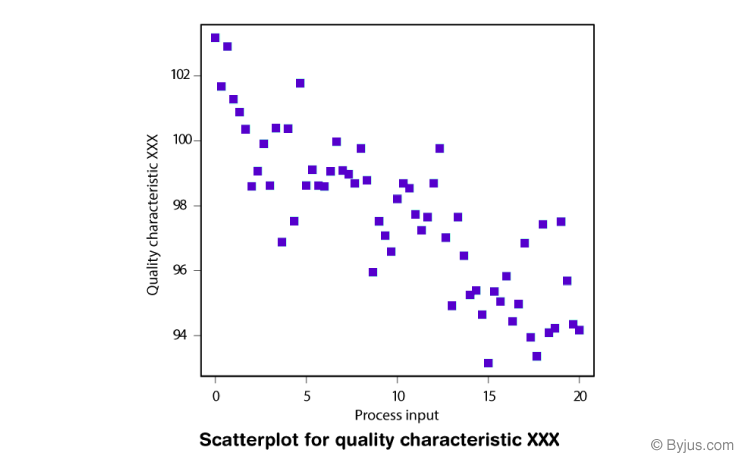# Bivariate Analysis

Bivariate analysis is one of the statistical analysis where two variables are observed. One variable here is dependent while the other is independent. These variables are usually denoted by X and Y. So, here we analyse the changes occured between the two variables and to what extent. Apart from bivariate, there are other two statistical analyses, which are Univariate (for one variable) and Multivariate (for multiple variables).

In statistics, we usually interpret the given set of data and make statements and predictions about it. During the research, an analysis attempts to determine the impact and cause in order to conclude the given variables.

## Definition of Bivariate Analysis

Bivariate analysis is stated to be an analysis of any concurrent relation between two variables or attributes. This study explores the relationship of two variables as well as the depth of this relationship to figure out if there are any discrepancies between two variables and any causes of this difference. Some of the examples are percentage table, scatter plot, etc.For analysis, it is necessary to recognise bivariate data first. Usually, the data comprises two measurements such as X and Y. For each measurement, the bivariate data can be interpreted as the pair (X, Y ). These variables are often called bivariate simple random sample (SRS). We can denote these variables as (X1,Y1), (X2,Y2),…..,(Xn,Yn). The bivariate data can be represented in a table as shown below :

 Observations X-Variable Y-Variable 1 10 5 2 5 4 3 6 3 4 8 2 5 4 -5

Note: The observations given in the table above can be independent of each other, but not the two measurements.

## Types of Bivariate Analysis

The types of a bivariate analysis will depend upon the types of variables or attributes we will use for analysing. The variable could be numerical, categorical or ordinal. If the independent variable is categorical, like a particular brand of pen, then logit or probit regression can be used. If independent and dependent both the attributes are ordinal, which means they have position or ranking, then we can measure a rank correlation coefficient. If dependent attribute is ordinal, then ordered logit or ordered probit can be utilised. Also, if the dependent attribute is either ratio or interval, like temperature scale, then we can measure regression. So based on these data, we can mention the types of bivariate data analysis:

1. Numerical and Numerical – In this type, both the variables of bivariate data, independent and dependent, are having numerical values.
2. Categorical and Categorical – When both the variables are categorical.
3. Numerical and Categorical – When one variable is numerical and one is categorical.

Download BYJU’S-The Learning App and learn the concepts with the help of personalised videos.